Courses

# NCERT Solutions Chapter 10 - Circles (II), Class 9, Maths Class 9 Notes | EduRev

Created by: Indu Gupta

## Class 9 : NCERT Solutions Chapter 10 - Circles (II), Class 9, Maths Class 9 Notes | EduRev

The document NCERT Solutions Chapter 10 - Circles (II), Class 9, Maths Class 9 Notes | EduRev is a part of Class 9 category.
All you need of Class 9 at this link: Class 9

Exercise 10.5

1. In Fig. 10.36, A,B and C are three points on a circle with centre O such that ∠BOC = 30° and ∠AOB = 60°. If D is a point on the circle other than the arc ABC, find ∠ADC.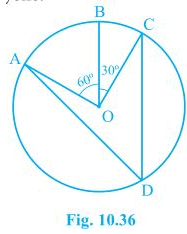Here,
∠AOC = ∠AOB + ∠BOC
⇒ ∠AOC = 60° + 30°
⇒ ∠AOC = 90°
We know that angle subtended by an arc at centre is double the angle subtended by it any point on the remaining part of the circle.
∠ADC = 1/2∠AOC = 1/2 × 90° = 45°

2. A chord of a circle is equal to the radius of the circle. Find the angle subtended by the chord at a point on the minor arc and also at a point on the major arc.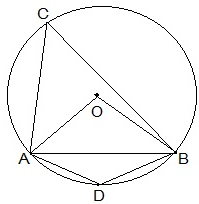Given,
AB is equal to the radius of the circle.
In ΔOAB,
OA = OB = AB = radius of the circle.
Thus, ΔOAB is an equilateral triangle.
∠AOC = 60°
also,
∠ACB = 1/2 ∠AOB = 1/2 × 60° = 30°
⇒ ∠ADB = 180° - 30° = 150°
Thus, angle subtend by the chord at a point on the minor arc and also at a point on the major arc are 150° and 30° respectively.

3. In Fig. 10.37, ∠PQR = 100°, where P, Q and R are points on a circle with centre O. Find ∠OPR.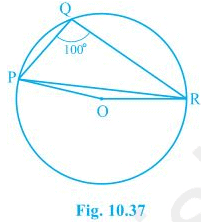Reflex ∠POR = 2 × ∠PQR = 2 × 100° = 200°
∴ ∠POR = 360° - 200° = 160°
In ΔOPR,
OP = OR (radii of the circle)
∠OPR = ∠ORP
Now,
∠OPR + ∠ORP +∠POR = 180° (Sum of the angles in a triangle)
⇒ ∠OPR + ∠OPR + 160° = 180°
⇒ 2∠OPR = 180° -  160°
⇒ ∠OPR = 10°

4. In Fig. 10.38, ∠ABC = 69°, ∠ ACB = 31°, find ∠BDC.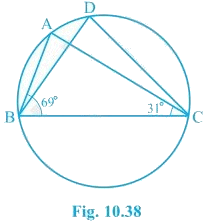∠BAC = ∠BDC (Angles in the segment of the circle)
In ΔABC,
∠BAC + ∠ABC + ∠ACB = 180° (Sum of the angles in a triangle)
⇒ ∠BAC + 69° + 31° = 180°
⇒ ∠BAC = 180° - 100°
⇒ ∠BAC = 80°
Thus, ∠BDC = 80°

5. In Fig. 10.39, A, B, C and D are four points on a circle. AC and BD intersect at a point E such that ∠BEC = 130° and ∠ECD = 20°. Find ∠ BAC.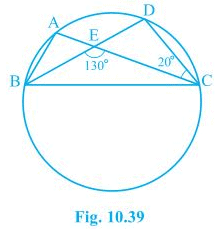∠BAC = ∠CDE (Angles in the segment of the circle)
In ΔCDE,
∠CEB = ∠CDE + ∠DCE (Exterior angles of the triangle)
⇒ 130° = ∠CDE + 20°
⇒ ∠CDE = 110°
Thus, ∠BAC = 110°

6. ABCD is a cyclic quadrilateral whose diagonals intersect at a point E. If ∠DBC = 70°, ∠BAC is 30°, find ∠BCD. Further, if AB = BC, find ∠ECD.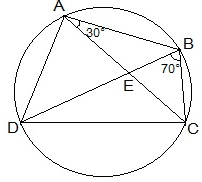For chord CD,
∠CBD = ∠CAD (Angles in same segment)
⇒ ∠BCD + 100° = 180°
⇒ ∠BCD = 80°
In ΔABC
AB = BC (given)
∠BCA = ∠CAB (Angles opposite to equal sides of a triangle)
∠BCA = 30°
also, ∠BCD = 80°
∠BCA + ∠ACD = 80°
⇒ 30° + ∠ACD = 80°
∠ACD = 50°
∠ECD = 50°

7. If diagonals of a cyclic quadrilateral are diameters of the circle through the vertices of the quadrilateral, prove that it is a rectangle.

Let ABCD be a cyclic quadrilateral and its diagonal AC and BD are the diameters of the circle through the vertices of the quadrilateral.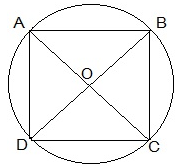∠ABC = ∠BCD = ∠CDA = ∠DAB = 90° (Angles in the semi-circle)
Thus, ABCD is a rectangle as each internal angle is 90°.

8. If the non-parallel sides of a trapezium are equal, prove that it is cyclic.

Given,
ABCD is a trapezium where non-parallel sides AD and BC are equal.
Construction,
DM and CN are perpendicular drawn on AB from D and C respectively.
To prove,
ABCD is cyclic trapezium.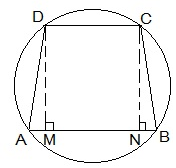Proof:
In ΔDAM and ΔCBN,
∠AMD = ∠BNC (Right angles)
DM = CN (Distance between the parallel lines)
ΔDAM ≅ ΔCBN by RHS congruence condition.
Now,
∠A = ∠B by CPCT
also, ∠B + ∠C = 180° (sum of the co-interior angles)
⇒ ∠A + ∠C = 180°
Thus, ABCD is a cyclic quadrilateral as sum of the pair of opposite angles is 180°.

9. Two circles intersect at two points B and C. Through B, two line segments ABD and PBQ are drawn to intersect the circles at A, D and P, Q respectively (see Fig. 10.40). Prove that ∠ACP = ∠QCD.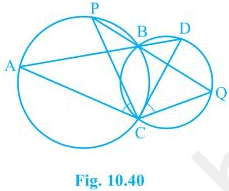Chords AP and DQ are joined.
For chord AP,
∠PBA = ∠ACP (Angles in the same segment) --- (i)
For chord DQ,
∠DBQ = ∠QCD (Angles in same segment) --- (ii)
ABD and PBQ are line segments intersecting at B.
∠PBA = ∠DBQ (Vertically opposite angles) --- (iii)
By the equations (i), (ii) and (iii),
∠ACP = ∠QCD

10. If circles are drawn taking two sides of a triangle as diameters, prove that the point of intersection of these circles lie on the third side.

Given,Two circles are drawn on the sides AB and AC of the triangle ΔABC as diameters. The circles intersected at D.

To prove,D lies on BC. We have to prove that BDC is a straight line.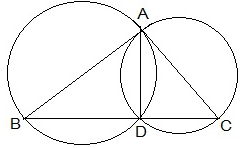Proof:
Now,
⇒ ∠BDC is straight line.
Thus, D lies on the BC.

11. ABC and ADC are two right triangles with common hypotenuse AC. Prove that ∠CAD = ∠CBD.

Given,
AC is the common hypotenuse. ∠B = ∠D = 90°.
To prove,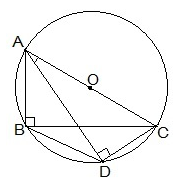Proof:
Since, ∠ABC and ∠ADC are 90°. These angles are in the semi circle. Thus, both the triangles are lying in the semi circle and AC is the diameter of the circle.
⇒ Points A, B, C and D are concyclic.
Thus, CD is the chord.
⇒ ∠CAD = ∠CBD (Angles in the same segment of the circle)

12. Prove that a cyclic parallelogram is a rectangle.

Given,
ABCD is a cyclic parallelogram.
To prove,
ABCD is rectangle.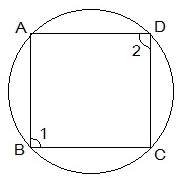Proof:
∠1 + ∠2 = 180° (Opposite angles of a cyclic parallelogram)
also, Opposite angles of a cyclic parallelogram are equal.
Thus,
∠1 = ∠2
⇒ ∠1 + ∠1 = 180°
⇒ ∠1 = 90°
One of the interior angle of the paralleogram is right angled. Thus, ABCD is a rectangle.

,

,

,

,

,

,

,

,

,

,

,

,

,

,

,

,

,

,

,

,

,

,

,

,

,

,

,

,

,

,

,

,

;